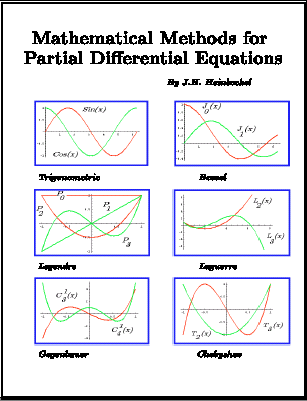Language/LinguaBooks of Mathematics· Book News · Most clicked · Least clicked · Books Index · Search on Amazon

Search for a BookPDE

 Analysis -> PDE Search on Amazon

A Partial Differential Equations Textbook
Author: J.H. Heinbockel  Language:Topics: Partial differential equations, Orthogonal functions, Fourier Series, Fourier Integrals, Separation of Variables, Boundary Value Problems, Laplace Transform, Fourier Transforms, Finit . . . . .
Lectures on Diffusion Problems and Partial Differential Equations
Author: S. R. S. Varadhan  Language:Contents: The Heat Equation; Kolmogorovs Theorem; The One Dimensional Random Walk; Construction of Wiener Measure: Generalised Brownian Motion; Markov Properties of Brownian Motion; Reflection Princi . . . . .
Beyond partial differential equations : A course on linear and quasi-linear abstract hyperbolic evolution equations
Author: Horst R. Beyer  Language:This course introduces the use of semigroup methods in the solution of linear and nonlinear (quasi-linear) hyperbolic partial differential equations, with particular application to wave equations and . . . . .
Hilbert Space Methods for Partial Differential Equations
Author: R. E. Showalter  Language:This book is an outgrowth of a course which we have given almost periodically over the last eight years. It is addressed to beginning graduate students of mathematics, engineering, and the physical sc . . . . .
Entropy and Partial Differential Equations
Author: Lawrence Craig Evans  Language:Contents: Entropy and equilibrium; Entropy and irreversibility; Continuum thermodynamics; Elliptic and parabolic equations; Conservation laws and kinetic equations; HamiltonJacobi and related equatio . . . . .
Partial Differential Equations An Introduction
Author: A.D.R. Choudary, Saima Parveen, Constantin Varsan  Language:Abstract: This book encompasses both traditional and modern methods treating partial differential equation (PDE) of first order and second order. There is a balance in making a selfcontained mathe . . . . .
Pseudodifferential Operators And Nonlinear PDE
Author: Michael E. Taylor  Language:Contents: Pseudodi®erential operators and linear PDE; Symbols with limited smoothness; Operator estimates and elliptic regularity; Paradifferential operators; Nonlinear hyperbolic systems; Propagation . . . . .
Partial Differential Equations and Complex Variable
Author: H C Rae  Language:Contents: Introduction; Revision of Complex Numbers; Functions of a Complex Variable; The elementary functions; Complex integration; Cauchys theorem; Calculus of residues; Origin of some PDE s of Mat . . . . .
Introduction to Classical Partial Differential Equations
Author: Horst Reinhard Beyer  Language:Contents: Conventions; Basic Definitions; Three Main Examples of PDE; First-Order Quasi-Linear PDE for One Real-Valued Function; Systems of Quasi-Linear PDE; Second-Order PDE for One Unknown Function; . . . . .
Introduction to Partial Differential Equations
Author: John Douglas Moore  Language:Contents: Power Series; Symmetry and Orthogonality; Fourier Series; Partial Differential Equations; PDEs in Higher Dimensions; Using Mathematica to solve differential equations.
Lectures on Partial Differential Equations and Representations of Semi-groups
Author: L. Schwartz  Language:Contents: Introduction; Mixed Problems in Partial Differential Equations; Vector valued Distributions; Vector valued Distributions; Spaces of distributions; The E-product of two locally convex Hausdor . . . . .
Computational Numerical Analysis of Partial Differential Equatio
Author: J. M. McDonough  Language:Contents: 1 Numerical Solution of Elliptic Equations; 2 Time-Splitting Methods for Evolution Equations; 3 Various Miscellaneous Topics.
Lectures on Elliptic Partial Differential Equations
Author: J.L. Lions   Language:In these lectures we study the boundary value problems associated with elliptic equation by using essentially L^2 estimates (or abstract analogues of such estimates. We consider only linear problem, . . . . .
Spectral Theory of Partial Differential Equations - Lecture Notes
Author: Richard S. Laugesen  Language:This mini-course of 20 lectures aims at highlights of spectral theory for self-adjoint partial differential operators, with a heavy emphasis on problems with discrete spectrum. Part I: Discrete Spectr . . . . .
Partial Differential Equations of Mathematical Physics
Author: William W. Syme  Language:Contents: Kinematics and Conservation; Strain; Ideal Fluids and the Euler Equations; Elastic Fluids and Acoustic Waves; Newtonian Fluids and the Navier-Stokes Equations; Linear Elasticity; Porous Flow . . . . .
Well-posedness and long time behavior in nonlinear dissipative hyperbolic-like evolutions with critical exponents
Author: Igor Chueshov, Irena Lasiecka  Language:These lectures present the analysis of stability and control of long time behavior of PDE models described by nonlinear evolutions of hyperbolic type. Specific examples of the models under considerati . . . . .
Introduction to the method of multiple scales
Author: Per Jakobsen  Language:These lecture notes give an introduction to perturbation method with main focus on the method of multiple scales as it applies to pulse propagation in nonlinear optics. The lecture notes are aimed at . . . . .
Exterior Differential Systems and Euler-Lagrange Partial Differe
Author: Robert L. Bryant, Phillip A. Griffiths, Daniel  Language:The first chapter contains an introduction of the classical Poincare-Cartan form in the context of EDS, followed by proofs of classical results, including a solution to the relevant inverse problem, . . . . .
Linear Methods of Applied Mathematics
Author: Evans M. Harrell II and James V. Herod  Language:This is a WWW textbook written by Evans M. Harrell II and James V. Herod, both of Georgia Tech. It is suitable for a first course on partial differential equations, Fourier series and special function . . . . .
Partial Differential Equations
Author: Prof. Jim Herod, Ret.  Language:This is a complete set of 40 Maple lessons for an undergraduate course in Partial Differential Equations. The course covers linear spaces; Fourier series; symbolic solutions to the heat, wave and Lapl . . . . .
Partial Differential Equations and the Method of Characteristics
Author: James V. Herod  Language:Contents: 1. Introduction to a Theme 2. PDE's Using ODE's 3. A Changing Population Hetrogeneous in Age 4. Stepping Up The Dimension 5. Complications: Nonlinear Equations 6. Shoc . . . . .

```Home |  Authors | About | Contact Us |  Email